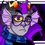# A Special Summation Problem

At math competitions, I often see a problem along the lines of "Let $S=\displaystyle\sum_{n=1}^\infty\dfrac{n^2}{2^n}$. Find $S.$" and notice that not that many people are able to solve it (the answer is $6$). I'm going to show how to solve that kind of problem in this note.

The first thing we need to do is to find the value of $\displaystyle\sum_{n=1}^\infty\dfrac{n}{k^n}$ for some $|k|>1.$ We can solve this with some clever manipulation and summation of geometric series. Recall that $\displaystyle\sum_{n=0}^\infty ab^n=\dfrac{a}{1-b}$ for $|b|<1.$

\begin{aligned} \displaystyle\sum_{n=1}^\infty\dfrac{n}{k^n}=\dfrac{1}{k}+\dfrac{2}{k^2}+\dfrac{3}{k^3}+\dfrac{4}{k^4}+&\ldots\\ =\dfrac{1}{k}+\dfrac{1}{k^2}+\dfrac{1}{k^3}+\dfrac{1}{k^4}+&\ldots\\ +\dfrac{1}{k^2}+\dfrac{1}{k^3}+\dfrac{1}{k^4}+&\ldots\\ +\dfrac{1}{k^3}+\dfrac{1}{k^4}+&\ldots\\ +\dfrac{1}{k^4}+&\ldots\\ &\ddots \end{aligned} We can see that each line we have created is a summable geometric series. Let's see what happens when we sum each line. Let $s_x=\displaystyle\sum_{n=x}^\infty\dfrac{1}{k^n}.$ \begin{aligned} s_1=\dfrac{1}{k}+\dfrac{1}{k^2}+\dfrac{1}{k^3}+\dfrac{1}{k^4}+\ldots&=\sum_{n=1}^\infty\dfrac{1}{k^n}=\dfrac{\frac{1}{k}}{1-\frac{1}{k}}=\dfrac{1}{k-1}\\ s_2=\dfrac{1}{k^2}+\dfrac{1}{k^3}+\dfrac{1}{k^4}+\ldots&=\sum_{n=2}^\infty\dfrac{1}{k^n}=\dfrac{\frac{1}{k^2}}{1-\frac{1}{k}}=\dfrac{1}{k^2-k}\\ s_3=\dfrac{1}{k^3}+\dfrac{1}{k^4}+\ldots&=\sum_{n=3}^\infty\dfrac{1}{k^n}=\dfrac{\frac{1}{k^3}}{1-\frac{1}{k}}=\dfrac{1}{k^3-k^2}\\ s_4=\dfrac{1}{k^4}+\ldots&=\sum_{n=4}^\infty\dfrac{1}{k^n}=\dfrac{\frac{1}{k^4}}{1-\frac{1}{k}}=\dfrac{1}{k^4-k^3}\\ &\vdots \end{aligned}

We can notice two things from this. First, our original problem can be rewritten as finding $\displaystyle\sum_{x=1}^\infty s_x.$ Also, $s_{x+1}=\dfrac{s_x}{k}.$ This means that $\{s_1, s_2, s_3\ldots\}$ is a geometric series. \begin{aligned} \sum_{x=1}^\infty s_x&=s_1+s_2+s_3+\ldots\\ &=\dfrac{1}{k-1}+\dfrac{1}{k^2-k}+\dfrac{1}{k^3-k^2}+\ldots\\ &=\dfrac{\frac{1}{k-1}}{1-\frac{1}{k}}\\ &=\dfrac{k}{(k-1)^2} \end{aligned}

So what does this mean for our original problem? After all, this only gives us a way to find $\displaystyle\sum_{n=1}^\infty\dfrac{n}{2^n},$ not $\displaystyle\sum_{n=1}^\infty\dfrac{n^2}{2^n}.$ Fortunately, we can make that leap with some nice index shifting.

First - what is index shifting? Index shifting is the technique of saying that $\displaystyle\sum_{i=a}^bx_i=\displaystyle\sum_{i=a-c}^{b-c}x_{i+c}.$ For example, $\displaystyle\sum_{i=1}^\infty\dfrac{1}{2^i}=\displaystyle\sum_{i=0}^\infty\dfrac{1}{2^{i+1}}.$ Now let's find a general formula for $S=\displaystyle\sum_{n=1}^\infty\dfrac{n^2}{k^n}$ to demonstrate how we can apply this technique.

\begin{aligned} \displaystyle\sum_{n=1}^\infty\dfrac{n^2}{k^n}&=\displaystyle\sum_{n=0}^\infty\dfrac{(n+1)^2}{k^{n+1}}\\ &=\displaystyle\sum_{n=0}^\infty\dfrac{n^2}{k^{n+1}}+\displaystyle\sum_{n=0}^\infty\dfrac{2n}{k^{n+1}}+\displaystyle\sum_{n=0}^\infty\dfrac{1}{k^{n+1}}\\ &=\displaystyle\sum_{n=1}^\infty\dfrac{n^2}{k^{n+1}}+\displaystyle\sum_{n=1}^\infty\dfrac{2n}{k^{n+1}}+\displaystyle\sum_{n=1}^\infty\dfrac{1}{k^n}\\ \end{aligned} That last step may seem a little strange, but take a look at what happens when $n=0$ in each sum.

In the first two sums, the $n=0$ term has a numerator of $0,$ meaning that the $n=0$ term is equal to $0.$ Thus, we can get rid of it and start the sum at the $n=1$ term. In the third sum, the $n=0$ term is not equal to $0,$ we can just index shift the $n=0$ out.

\begin{aligned} \displaystyle\sum_{n=1}^\infty\dfrac{n^2}{k^{n+1}}+\displaystyle\sum_{n=1}^\infty\dfrac{2n}{k^{n+1}}+\displaystyle\sum_{n=1}^\infty\dfrac{1}{k^n}&=\dfrac{1}{k}\displaystyle\sum_{n=1}^\infty\dfrac{n^2}{k^n}+\dfrac{2}{k}\displaystyle\sum_{n=1}^\infty\dfrac{n}{k^n}+\displaystyle\sum_{n=1}^\infty\dfrac{1}{k^n} \end{aligned} We have simplified the problem enough to solve for $S$. \begin{aligned} S&=\dfrac{S}{k}+\dfrac{2}{k}\times\dfrac{k}{(k-1)^2}+\dfrac{1}{k-1}\\ S\left(\dfrac{k-1}{k}\right)&=\dfrac{2}{(k-1)^2}+\dfrac{k-1}{(k-1)^2}\\ &=\dfrac{k+1}{(k-1)^2}\\ S&=\dfrac{k(k+1)}{(k-1)^3} \end{aligned} You can use similar techniques to find higher order sums, though problems going past $\displaystyle\sum_{n=1}^\infty\dfrac{n^4}{k^n}$ are trolls.

Here is a helpful list for sums of order $\leq6$

\begin{aligned} \sum_{n=1}^\infty\dfrac{1}{k^n}&=\dfrac{1}{k-1}\\ \sum_{n=1}^\infty\dfrac{n}{k^n}&=\dfrac{k}{(k-1)^2}\\ \sum_{n=1}^\infty\dfrac{n^2}{k^n}&=\dfrac{k(k+1)}{(k-1)^3}\\ \sum_{n=1}^\infty\dfrac{n^3}{k^n}&=\dfrac{k\left(k^2+4k+1\right)}{(k-1)^4}\\ \sum_{n=1}^\infty\dfrac{n^4}{k^n}&=\dfrac{k\left(k^3+11k^2+11k+1\right)}{(k-1)^5}\\ \sum_{n=1}^\infty\dfrac{n^5}{k^n}&=\dfrac{k\left(k^4+26k^3+66k^2+26k+1\right)}{(k-1)^6}\\ \sum_{n=1}^\infty\dfrac{n^6}{k^n}&=\dfrac{k\left(k^5+57k^4+302k^3+302k^2+57k+1\right)}{(k-1)^7}\\ \end{aligned} It should be noted that $\displaystyle\sum_{n=1}^\infty\dfrac{n^x}{k^n}=\dfrac{kA_n(k)}{(k-1)^{x+1}}$ where $A_n(k)$ is the $n$th Eulerian polynomial.

I hope this helped you out! Best of luck solving these kinds of problems!Note by Trevor B.
6 years, 3 months ago

This discussion board is a place to discuss our Daily Challenges and the math and science related to those challenges. Explanations are more than just a solution — they should explain the steps and thinking strategies that you used to obtain the solution. Comments should further the discussion of math and science.

When posting on Brilliant:

• Use the emojis to react to an explanation, whether you're congratulating a job well done , or just really confused .
• Ask specific questions about the challenge or the steps in somebody's explanation. Well-posed questions can add a lot to the discussion, but posting "I don't understand!" doesn't help anyone.
• Try to contribute something new to the discussion, whether it is an extension, generalization or other idea related to the challenge.
• Stay on topic — we're all here to learn more about math and science, not to hear about your favorite get-rich-quick scheme or current world events.

MarkdownAppears as
*italics* or _italics_ italics
**bold** or __bold__ bold
- bulleted- list
• bulleted
• list
1. numbered2. list
1. numbered
2. list
Note: you must add a full line of space before and after lists for them to show up correctly
paragraph 1paragraph 2

paragraph 1

paragraph 2

[example link](https://brilliant.org)example link
> This is a quote
This is a quote
    # I indented these lines
# 4 spaces, and now they show
# up as a code block.

print "hello world"
# I indented these lines
# 4 spaces, and now they show
# up as a code block.

print "hello world"
MathAppears as
Remember to wrap math in $$ ... $$ or $ ... $ to ensure proper formatting.
2 \times 3 $2 \times 3$
2^{34} $2^{34}$
a_{i-1} $a_{i-1}$
\frac{2}{3} $\frac{2}{3}$
\sqrt{2} $\sqrt{2}$
\sum_{i=1}^3 $\sum_{i=1}^3$
\sin \theta $\sin \theta$
\boxed{123} $\boxed{123}$

## Comments

Sort by:

Top Newest

Check this PolyLogarithm

- 6 years, 2 months ago

Log in to reply

That's simply Brilliant , Trevor

- 6 years, 3 months ago

Log in to reply

Thanks @Azhaghu Roopesh M! I'm glad it helps.

- 6 years, 3 months ago

Log in to reply

Nice algebraic method; I mostly use calculus for these sorts but this was an eye-opener.

- 6 years, 3 months ago

Log in to reply

Very nice note!

- 6 years, 2 months ago

Log in to reply

Amazing Man !!!

- 6 years, 2 months ago

Log in to reply

These questions are what I call Arithmetic Geometric Progression. I started a wiki page on it, but it is still a work in progress. Help would be appreciated!

Staff - 6 years, 2 months ago

Log in to reply

@Trevor B. We've made several edits to the Arithmetic-Geometric Progression wiki page. Can you look over it and see if there are any improvements?

Staff - 5 years, 10 months ago

Log in to reply

Thanks a lot for the note. It helped a lot.

- 5 years, 11 months ago

Log in to reply

Thanks!!!!!!

- 5 years, 10 months ago

Log in to reply

×

Problem Loading...

Note Loading...

Set Loading...